# Quiz Discussion

The least perfect square number divisible by 3, 4, 5, 6 and 8 is = ?

Course Name: Quantitative Aptitude

• 1] 900
• 2] 1200
• 3] 2500
• 4] 3600
##### Solution
No Solution Present Yet

#### Top 5 Similar Quiz - Based On AI&ML

Quiz Recommendation System API Link - https://fresherbell-quiz-api.herokuapp.com/fresherbell_quiz_api

# Quiz
1
Discuss

What percentage of the numbers from 1 to 50 have squares that end in the digit 1 ?

• 1] 1%
• 2] 5%
• 3] 10%
• 4] 11%
• 5] 20%
##### Solution
2
Discuss

Solved $$\root 4 \of {{{\left( {625} \right)}^3}} = ?$$

• 1]

$$\root 3 \of {1875}$$

• 2]

25

• 3]

125

• 4]

None of these

##### Solution
3
Discuss

Which number can replace both the question marks in the equation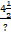=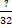?

• 1]

12

• 2]

7

• 3]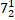• 4]

None of these

##### Solution
4
Discuss

The least number of 4 digits which is a perfect square is = ?

• 1] 1000
• 2] 1016
• 3] 1024
• 4] 1036
##### Solution
5
Discuss

One-fourth of the sum of prime numbers, greater than 4 but less than 16, is the square of = ?

• 1] 3
• 2] 4
• 3] 5
• 4] 7
##### Solution
6
Discuss

Value of $$\sqrt {0.01} \times \root 3 \of {0.008} - 0.02$$    is = ?

• 1] 0
• 2] 1
• 3] 2
• 4] 3
##### Solution
7
Discuss

Three fifth of the square of a certain number is 126.15, What is the number?

• 1]

210.25

• 2]

75.69

• 3]

14.5

• 4]

145

##### Solution
8
Discuss

R is a positive number. It is multiplied by 8 and then squared. The square is now divided by 4 and the square root is taken. The result of the square root is Q. What is the value of Q ?

• 1] 3R
• 2] 4R
• 3] 7R
• 4] 9R
##### Solution
9
Discuss

$$\left( {\frac{{2 + \sqrt 3 }}{{2 - \sqrt 3 }} + \frac{{2 - \sqrt 3 }}{{2 + \sqrt 3 }} + \frac{{\sqrt 3 - 1}}{{\sqrt 3 + 1}}} \right)$$     simplifies to = ?

• 1]

$$16 - \sqrt 3$$

• 2]

$$4 - \sqrt 3$$

• 3]

$$2 - \sqrt 3$$

• 4]

$$2 + \sqrt 3$$

##### Solution
10
Discuss

If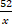=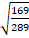the value of x is = ?

• 1] 52
• 2] 58
• 3] 62
• 4] 68
# Quiz